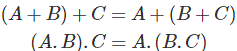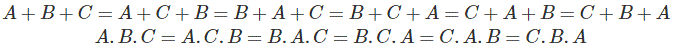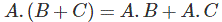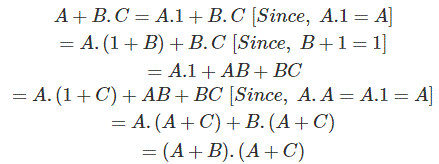Notes on Laws of Boolean Algebra and De Morgan's Theorem | Grade 11 > Computer Science > Logic Function and Boolean Algebra | KULLABS.COM

Notes, Exercises, Videos, Tests and Things to Remember on Laws of Boolean Algebra and De Morgan's Theorem

Please scroll down to get to the study materials.

• Note
• Things to remember

### LAW OF BOOLEAN ALGEBRA

As 0 and 1 are logic symbol that is used to represent a digital output or input that are used as constants permanently for “Open"

or “Closed” circuit rules. Boolean Algebra expression have been invented to help to reduce the number of logic gates that is used to perform a particular logic operation resulting a list of theorems or functions commonly knownas the "Laws of Boolean Algebra".

Boolean algebra was invented by world famous mathematician George Boole, in 1854. He published it in his book named "An Investigation of the Laws of Thought". There are theorems of these boolean that are used to make calculation fastest and easier ever than ever.

Boolean Algebra is Mathematics, that is used to analyze digital gates and circuits. We can use “Laws of Boolean” to reduce and simplify a complex Boolean expression to reduce the number of logic gates.

• Associative Laws for Boolean Algebra

This law is for several variables, where the OR operation of the variable result is same though the grouping of the variables.This law is quite same in the case of AND operators.• Cumulative Law for Boolean AlgebraAccording to Cumulative Law, the order of OR operations and AND operations conducted on the variables make no difference.

Hence, the values fo A + B and B + A are both equal.

• Distributive Laws for Boolean Algebra

This law can be composed of two operators: AND and OR.

Hence,the values of A . ( B + C ) and A. B + A . C are equal.Proof:• Identity Law for Boolean algebra

A term ORed with a "0" or AND with a "1" will always equal that term.Hence, the output will be same as the input.• #### Complements laws of Boolean algebra

A term ANDed with its complement equal "0" and a term OR`ed with its complement equals "1".Hence, the sum of an input and its complement will always be true. (Leon and Leon)#### Basic Identities of Boolean Algebra#### De Morgan’s Theorem:

De Morgan's theorem is associated with Boolean algebra, which was given by great logical and mathematician, De Morgan. So, it is called "De Morgan's theorem".

It consists of first and second theorem which are described below:

• #### First Theorem:

The De Morgan’s first theorem states, “The complement of the sum is equal to the product of complement of individual variable”. Let X and Y be two Boolean variables then De Morgan’s theorem mathematically expressed as (X + Y)l = Xl . Yl

Proof:

• #### Second Theorem:

De Morgan’s second theorem states,” The complement of a product is equal to the sum of the complements of individual variable”. Let X and Y be two Boolean variables then De Morgan’s theorem mathematically expressed as (X . Y)l = Xl + Yl

Proof:De-Morgan's laws can also be implemented in Boolean algebra in the following steps:-

1. While using Boolean algebra, firstly, we exchange the given operator like if (+) is there then exchange it with (.) and if (.) is there then exchange it with (+).
2. Next, find the complement of each term.

De-Morgan's theorem can be proved by the simple induction method from the table given below.

 1 2 3 4 5 6 7 8 9 10 X Y X’ Y' X+Y X.Y (X+Y)’ X’.Y’ (X.Y)’ X’+Y’ 0 0 1 1 0 0 1 1 1 1 0 1 1 0 1 0 0 0 1 1 1 0 0 1 1 0 0 0 1 1 1 1 0 0 1 1 0 0 0 0

From the above table, firstly, the value of X= 0 and the value of Y = 0. Now, for this values X' = 1, Y' = 1. Again, X+Y = 0 and X.Y = 0. Thus (X+Y)' = 1 and (X.Y)' = 1, X' + Y' = 1 and X'.Y' = 1. From this table, we can see that the value of column no. 7 and 8 are equal and column no. 9 and 10 are also equal which proves the De-Morgan's theorem. Again, different values of X and Y, we see the same thing i.e. column no. 7 and 8 are equal to each other and column no. 9 and 10 are equal to each other. Thus, the above table proves De-Morgan's theorem.

(Shrestha, Manandhar, and Roshan) (Leon and Leon) (electrical4u)

### Bibliography

electrical4u. 2014-2016. 06 June 2016. <http://www.electrical4u.com/de-morgans-theorem-and-demorgans-laws/>.
Leon, Alex and Mathews Leon. Fundamental of Information Technology. India, 2015.
Shrestha, Prachanda Ram, et al. Computer Essentials. Kathmandu: Asmita's Publication, 2014.

• Boolean Algebra is Mathematics that is used to analyze digital gates and circuits.
• We can use “Laws of Boolean” to reduce and simplify a complex
• Boolean expression to reduce the number of logic gates
.